# Calculus 3 : Distance between Vectors

## Example Questions

1 2 3 5 Next →

### Example Question #41 : Distance Between Vectors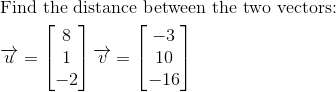Possible Answers: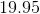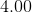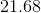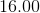Correct answer:Explanation: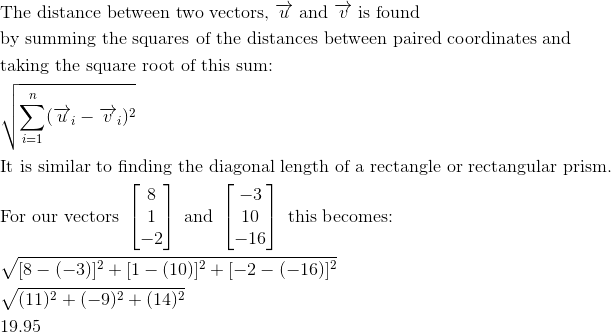### Example Question #42 : Distance Between Vectors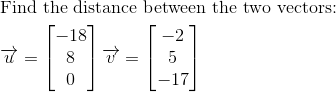Possible Answers: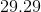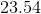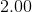Correct answer:Explanation: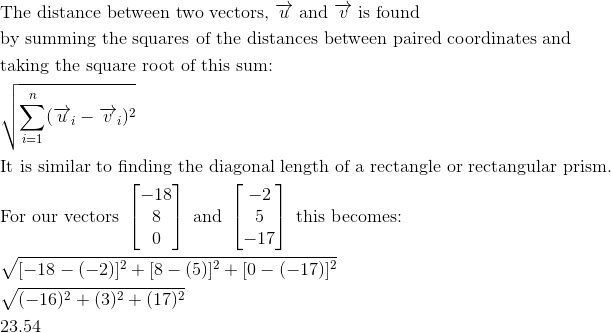### Example Question #43 : Distance Between Vectors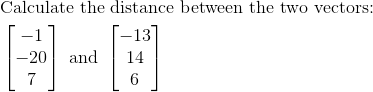Possible Answers: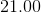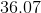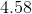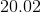Correct answer:Explanation: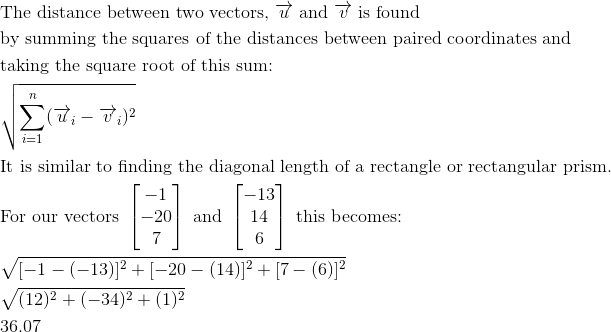### Example Question #44 : Distance Between Vectors

Find the distance between the vectors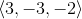and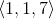Possible Answers: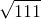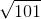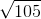Correct answer:Explanation:

To find the distance between two vectors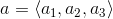and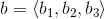, you use the formula: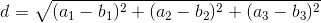Using the vectors from the problem statement, we get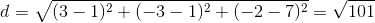### Example Question #45 : Distance Between Vectors

Find the distance between the vectors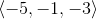and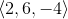Possible Answers: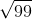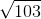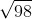Correct answer:Explanation:

To find the distance between two vectorsand, you use the formula:Using the vectors from the problem statement, we get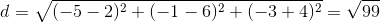1 2 3 5 Next →

### All Calculus 3 Resources